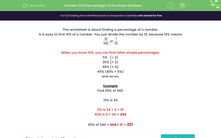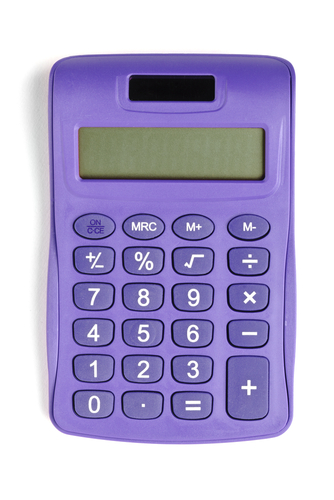# Find Percentages of Given Numbers

In this worksheet, students will find a percentage of a given number.Key stage:  KS 3

Curriculum topic:   Number

Curriculum subtopic:   Define, Interpret and Compare Percentages

Difficulty level:#### Worksheet Overview

This activity is about finding percentages of numbers.

It is easy to find 10% of any number because 10% = 10/100 = 1/10

Simply divide by 10:

So 10% of 192 is 19.2

10% of 54 g is 5.4 g etc.

When you know 10%, it is easy to find 1% - simply divide by 10 again!

To find 2%, we can find 1% and multiply by 2.

To find 5%, we can find 10% and divide by 2.

To find 30%, we can find 10% and multiply by 3.

We can then add any combinations together!

Example

Find 65% of 340.

10% is 34

So, 60% is 6 × 34 = 204

5% is 34 ÷ 2 = 17

65% = 204 + 17 = 221

You can work out percentages on a calculator either by using the % key, or by multiplying the number by the required percentage and dividing by 100.For this activity, we will be using the method above, so no calculators allowed!!

Let's get started on the questions.

### What is EdPlace?

We're your National Curriculum aligned online education content provider helping each child succeed in English, maths and science from year 1 to GCSE. With an EdPlace account you’ll be able to track and measure progress, helping each child achieve their best. We build confidence and attainment by personalising each child’s learning at a level that suits them.

Get started